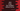# Java program to swap two numbers without using a third number## Java program to swap two numbers without using a third number:

In this post, we will learn how to swap two numbers without using a third number or temporary number. We can easily swap two numbers if we have a third number. We have to follow the below steps for that:

• Store the value of the first variable in the temp variable.
• Store the value of the second variable in the first variable.
• Store the value of the temp variable in the second variable.

It will swap the values in the first and the second variable.

But, if we want to swap two numbers without using a third variable, we need to follow a different algorithm. In this post, we will learn how to swap two numbers without using a third variable in Java.

### Algorithm to follow:

We will use the below algorithm to swap two numbers without using a third temporary variable:

For example, if the numbers are firstNumber and secondNumber, we can follow the below steps to swap these:

• firstNumber = firstNumber + secondNumber
• secondNumber = firstNumber - secondNumber
• firstNumber = firstNumber - secondNumber

For example, if firstNumber is 33, and secondNumber is 44,

• firstNumber = firstNumber + secondNumber = 33 + 44 = 77
• secondNumber = firstNumber - secondNumber = 77 - 44 = 33
• firstNumber = firstNumber - secondNumber = 77 - 33 = 44

So, both are swapped.

### Java program:

Let’s write down the Java program:

``````import java.util.Scanner;

class Main {
public static void main(String[] args) {
int first, second;
Scanner sc = new Scanner(System.in);

System.out.println("Enter the first number: ");
first = sc.nextInt();

System.out.println("Enter the second number: ");
second = sc.nextInt();

System.out.println("First number: " + first + ", Second number: " + second);

first = first + second;
second = first - second;
first = first - second;

System.out.println("After the swap: ");
System.out.println("First number: " + first + ", Second number: " + second);
}
}``````

Here,

• first and second are two integer variables to hold the first and the second numbers.
• sc is a Scanner variable to read the user content.
• We are asking the user to enter the numbers and by using the scanner variable, we are reading the numbers. These are stored in the first and secondvariables.
• We are using the same three steps to swap the values of these variables.
• The values are printed before and after the swap is done.

If you run this program, it will give output as like below:

``````Enter the first number:
13
Enter the second number:
14
First number: 13, Second number: 14
After the swap:
First number: 14, Second number: 13``````

As you can see here, the numbers are swapped.